### Home > INT2 > Chapter 10 > Lesson 10.1.3 > Problem10-36

10-36.

Coach Daniele recorded the 5K race times of each member of her cross country team along with the number of kilometers they ran in training during previous week. She is hoping to use the information to establish team goals for the coming season.

Coach Daniele has used technology to come up with the following regression equations and plots, but now she needs your help. For each equation, $x$ represents the number of kilometers run in training during the previous week and $y$ represents the 5K race times in minutes.

Regression Equation

Scatterplot

Residual Plot

1: $y=16.0+10(0.95)^x$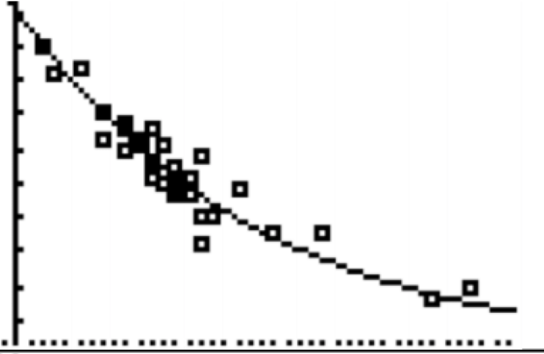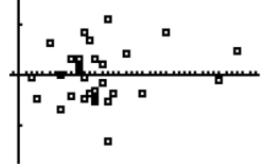2: $y=0.0056x^2-0.413x+25.58$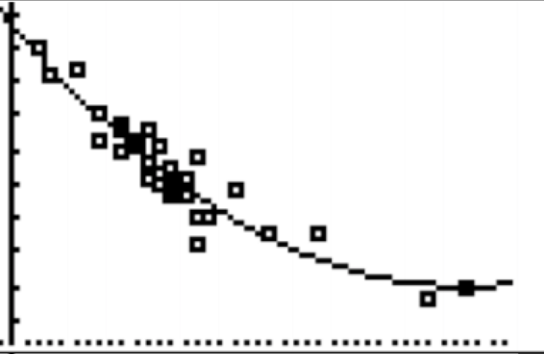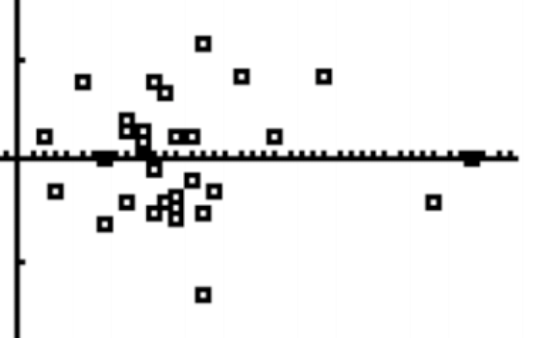3: $y=24.0-0.1972x$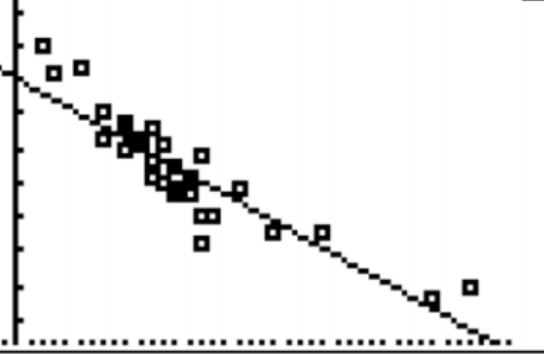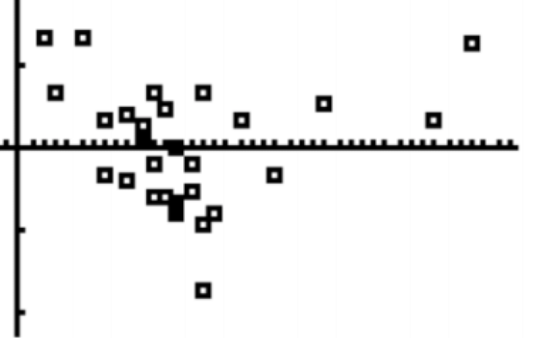1. Determine the type of each equation for Coach Daniele (linear, exponential, etc.).

Look at each equation.

2. Explain to Coach Daniele which model you believe is the best and why.

The exponential most closely models the data.

3. Use the model you chose in part (b) to predict a 5K time for athletes who train $10, 20,$ and $30$ km a week. Show your work.

Substitute each distance into the best equation.

4. Coach Daniele would like all of her varsity athletes to run the 5K in under $20$ minutes so she solves the equation $20 = 16 + 10(0.95)^x$ and determines that $x ≈ 17.86$ km. Coach Daniele now proudly proclaims that if she makes her athletes run $18$ km per week they should run sub $20$-minute 5K races. Assuming that her arithmetic is correct, explain the flaw(s) in her plan.

Does this study show cause and effect?